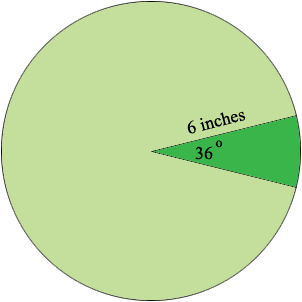SEARCH HOMEMath Central Quandaries & QueriesQuestion from trisha, a student: Given a circle with a radius of 6 inches. Find the area of the sector that is formed by an arc of 36 degrees. Round your answer to the nearest tenth of an inch.Hi Trisha,One complete revolution of the circle is 360o and hence the 36o angle is one tenth of the way around the circle. The symmetry of the circle then ensures that the area of the sector is one tenth the area of the circle.

I hope this helps,
PennyMath Central is supported by the University of Regina and The Pacific Institute for the Mathematical Sciences.